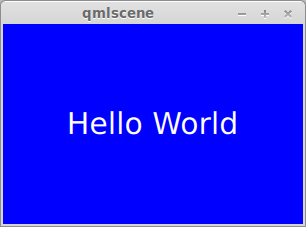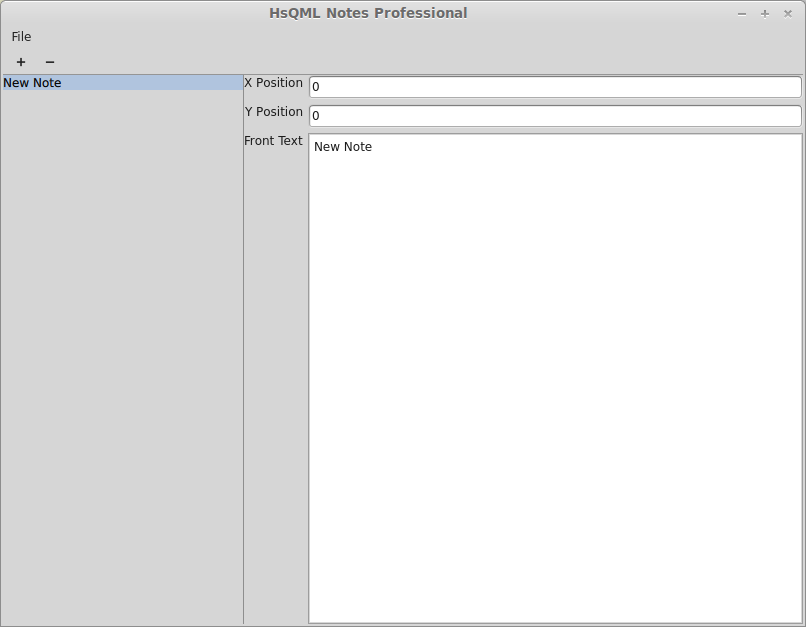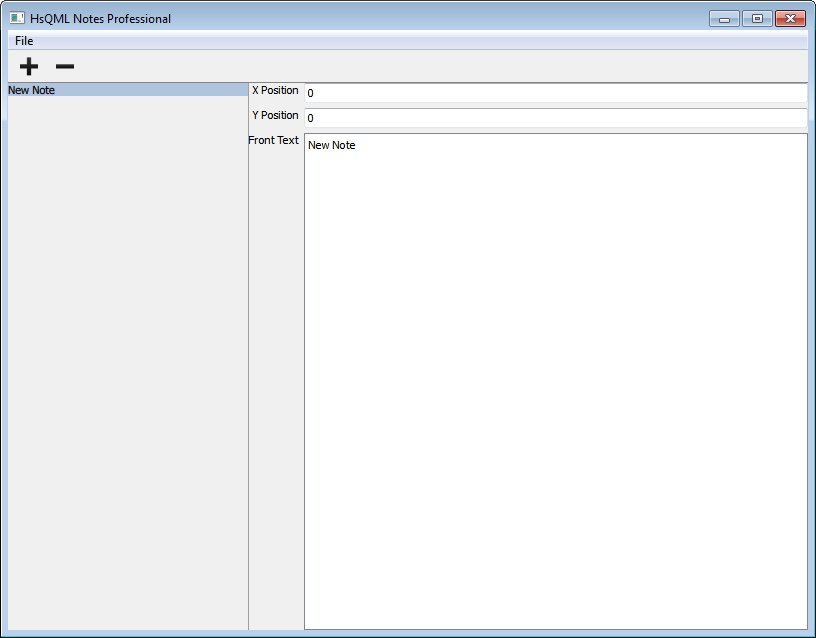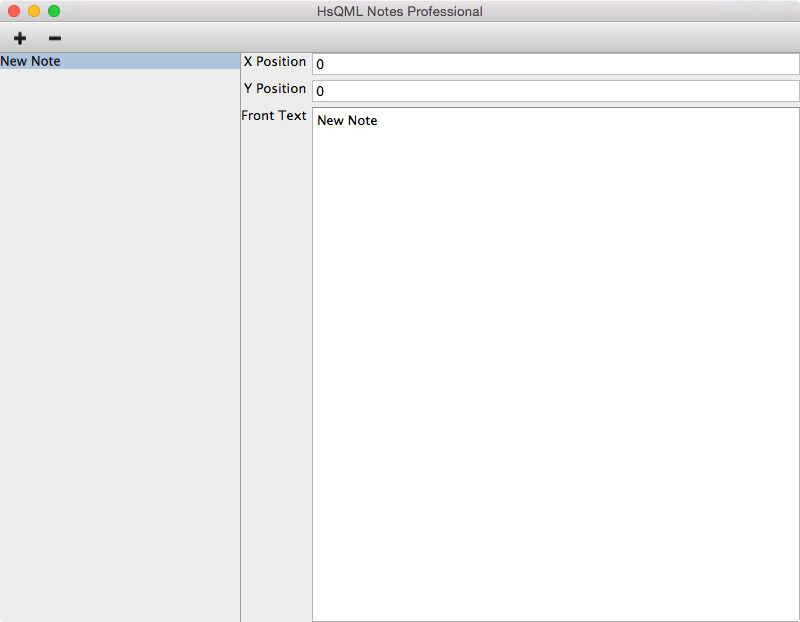Building Pragmatic User Interfaces in Haskell with HsQML

# QML /= JavaScript

## QML Example

``````
import QtQuick 2.0

Rectangle {
width: 300; height: 200;
color: 'blue';

Text {
anchors.centerIn: parent;
color: 'white'; font.pixelSize: 30;
text: 'Hello World';
}
}
``````

## QML Example in Action# Let's build an application in Haskell!

## 😃# Data Model

Field NameData Type
idINTEGER PRIMARY KEY
xINTEGER
yINTEGER
frontTEXT

## Prelude to Action

``````
{-# LANGUAGE DeriveDataTypeable #-}
module Main where

import Data.Text (Text)
import qualified Data.Text as T
import Data.Typeable (Typeable)

import qualified Database.SQLite.Simple as S
import qualified Database.SQLite.Simple.FromField as S
import qualified Database.SQLite.Simple.ToField as S

import Graphics.QML
``````

## Create Table

``````
createTable :: S.Connection -> IO ()
createTable conn =
S.execute_ conn . S.Query . T.pack \$
"CREATE TABLE IF NOT EXISTS notes (" ++
"id INTEGER PRIMARY KEY AUTOINCREMENT, " ++
"x INTEGER, y INTEGER, front TEXT)"
``````

## Data type representing a Note

``````
newtype Note = Note {noteId :: Int} deriving (Eq, Ord, Typeable)
``````

## Select and fold over Notes in database

``````
selectNotes :: S.Connection -> a -> (a -> Note -> IO a) -> IO a
selectNotes conn zero func =
let query = S.Query \$ T.pack
"SELECT id FROM notes ORDER BY id DESC"
in S.fold_ conn query zero (\acc (S.Only i) -> func acc \$ Note i)
``````

## Insert new Note in database

``````
insertNote :: S.Connection -> Int -> Int -> Text -> IO ()
insertNote conn x y front =
S.execute conn (S.Query \$ T.pack
"INSERT INTO notes (x, y, front) VALUES (?, ?, ?)")
(x, y, front)
``````

## Delete Note from database

``````
deleteNote :: S.Connection -> Note -> IO ()
deleteNote conn =
let query = S.Query \$ T.pack "DELETE FROM notes WHERE id = ?"
in S.execute conn query . S.Only . noteId
``````

## Read Note field from database

``````
readNoteAttrib :: (S.FromField a) => S.Connection ->
String -> ObjRef Note -> IO a
readNoteAttrib conn attrib note = do
let query = S.Query . T.pack \$
"SELECT " ++ attrib ++ " FROM notes WHERE id = ?"
[S.Only value] <- S.query conn query (
S.Only . noteId \$ fromObjRef note)
return value
``````

## Update Note field in database

``````
writeNoteAttrib :: (S.ToField a) => S.Connection ->
String -> SignalKey (IO ()) -> ObjRef Note -> a -> IO ()
writeNoteAttrib conn attrib changeKey note value = do
let query = S.Query . T.pack \$
"UPDATE notes SET " ++ attrib ++ " = ? WHERE id = ?"
S.execute conn query (value, noteId \$ fromObjRef note)
``````    fireSignal changeKey note
``````

# Define Classes

``````
data Class tt

newClass :: forall tt. Typeable tt => [Member tt] -> IO (Class tt)
``````

# Define Methods

``````
defMethod :: forall tt ms.
(Marshal tt, CanGetFrom tt ~ Yes, IsObjType tt ~ Yes,
MethodSuffix ms) =>
````    String -> (tt -> ms) -> Member (GetObjType tt)````
• (tt -> ms) is the callback which implements the method.
• (tt -> ms) is a variadic function.
• (tt -> ms) lives in the IO monad.
``````
class MethodSuffix a

instance (Marshal a, CanGetFrom a ~ Yes, MethodSuffix b) =>
MethodSuffix (a -> b)

instance (Marshal a, CanReturnTo a ~ Yes) =>
MethodSuffix (IO a)
``````

``````
ObjRef MyObject -> Int -> Text -> IO Bool
``````

# =

``````
(Marshal tt, CanGetFrom tt ~ Yes, IsObjType tt ~ Yes,
MethodSuffix ms) => tt -> ms
``````
``````
(Marshal (ObjRef MyObject), CanGetFrom (ObjRef MyObject) ~ Yes,
IsObjType (ObjRef MyObject) ~ Yes)

ObjRef MyObject -> ms
``````
``````
(Marshal a, CanGetFrom a ~ Yes, MethodSuffix b) => (a -> b)

ObjRef MyObject -> arg0 -> ms
``````
``````
(Marshal Int, CanGetFrom Int ~ Yes)

ObjRef MyObject -> Int -> ms
``````
``````
(Marshal a, CanGetFrom a ~ Yes, MethodSuffix b) => (a -> b)

ObjRef MyObject -> Int -> arg1 -> ms
``````
``````
(Marshal Text, CanGetFrom Text ~ Yes)

ObjRef MyObject -> Int -> Text -> ms
``````
``````
(Marshal Bool, CanReturnTo Bool ~ Yes)

ObjRef MyObject -> Int -> Text -> IO Bool
``````

# Define Signals

## Signals are "inverse methods"

``````
defSignal :: forall obj skv.
(SignalKeyValue skv) => String -> skv -> Member obj
``````
``````
data SignalKey p

instance (SignalSuffix p) => SignalKeyValue (SignalKey p)
``````

# Fire Signals

## QML data-bindings can attach to signals and listen for events

``````
fireSignal ::
forall tt skv. (Marshal tt, CanPassTo tt ~ Yes,
IsObjType tt ~ Yes, SignalKeyValue skv) =>
``````        skv -> tt -> SignalValueParams skv
``````

## Underneath all the type machinary,

• fireSignal is a variadic function.
• fireSignal lives in the IO monad.
``````
class SignalSuffix ss
``````

# Define Properties

## There are lots of different kinds of property you can define.

``````
defPropertyConst :: forall tt tr.
(Marshal tt, CanGetFrom tt ~ Yes, IsObjType tt ~ Yes, Marshal tr,
CanReturnTo tr ~ Yes) => String ->
(tt -> IO tr) -> Member (GetObjType tt)
``````

# Exciting Properties

``````
defPropertySigRW :: forall tt tr skv.
(Marshal tt, CanGetFrom tt ~ Yes, IsObjType tt ~ Yes,
Marshal tr, CanReturnTo tr ~ Yes, CanGetFrom tr ~ Yes,
SignalKeyValue skv) => String ->
````    skv ->``    (tt -> IO tr) ->``    (tt -> tr -> IO ()) ->````    Member (GetObjType tt)
``````

## A SigRW property can:

• Can be written to
• Can signal changes asynchronously

# Create Objects

``````
data ObjRef tt

newObject :: forall tt. Class tt -> tt -> IO (ObjRef tt)
``````

## An ObjRef wraps a Haskell value

``````
fromObjRef :: ObjRef tt -> tt
``````

# Let's try that again...

``````
writeNoteAttrib :: (S.ToField a) => S.Connection ->
````    String -> SignalKey (IO ()) -> ObjRef Note -> a -> IO ()````writeNoteAttrib conn attrib changeKey note value = do
let query = S.Query . T.pack \$
"UPDATE notes SET " ++ attrib ++ " = ? WHERE id = ?"
S.execute conn query (value, noteId \$ fromObjRef note)
``````    fireSignal changeKey note
``````

# The Big Picture## Building the Note object

``````
createContext :: S.Connection -> IO (ObjRef ())
createContext conn = do
````    changeKey <- newSignalKey``    noteClass <- newClass [````       defPropertySigRW "x" changeKey
(readNoteAttrib conn "x" :: ObjRef Note -> IO Int)
(writeNoteAttrib conn "x" changeKey),
``````        defPropertySigRW "y" changeKey
(readNoteAttrib conn "y" :: ObjRef Note -> IO Int)
(writeNoteAttrib conn "y" changeKey),``````        defPropertySigRW "front" changeKey
(readNoteAttrib conn "front" :: ObjRef Note -> IO Text)
(writeNoteAttrib conn "front" changeKey)]
...
``````
1. Creates a new SignalKey  [:: SignalKey (IO ())]
2. Defines the class for the Note object  [:: Class Note]
3. Defines a property backed by the database  [:: Member Note]

## UML again## Building the Context object (I)

``````
createContext :: S.Connection -> IO (ObjRef ())
createContext conn = do
...
````    notePool <- newFactoryPool (newObject noteClass)````    rootClass <- newClass [
defPropertySigRO' "notes" changeKey (\_ ->
selectNotes conn [] (\objs note -> do````                object <- getPoolObject notePool note````                return \$ object:objs)),
...
``````
1. Create a FactoryPool  [:: FactoryPool Note]
2. Get an object from the pool  [:: ObjRef Note]

# FactoryPools

``````
data FactoryPool tt

newFactoryPool :: (Ord tt) =>
(tt -> IO (ObjRef tt)) -> IO (FactoryPool tt)

getPoolObject :: (Ord tt) =>
FactoryPool tt -> tt -> IO (ObjRef tt)
``````
• It's essentially a Map from tt to ObjRef tt
• Except it can purge ObjRefs which aren't being used any more
• You could just track the ObjRefs yourself, but this is easier

## Building the Context object (I*)

``````
newtype Note = Note {noteId :: Int} deriving (Eq, Ord, Typeable)
``````
``````
createContext :: S.Connection -> IO (ObjRef ())
createContext conn = do
...
````    notePool <- newFactoryPool (newObject noteClass)````    rootClass <- newClass [
defPropertySigRO' "notes" changeKey (\_ ->
selectNotes conn [] (\objs note -> do````                object <- getPoolObject notePool note````                return \$ object:objs)),
...
``````
1. Create a FactoryPool  [:: FactoryPool Note]
2. Get an object from the pool  [:: ObjRef Note]

## Building the Context object (II)

``````
createContext :: S.Connection -> IO (ObjRef ())
createContext conn = do
...
rootClass <- newClass [
...
defMethod' "insertNote" (\this x y front -> do
insertNote conn x y front
fireSignal changeKey this),
defMethod' "deleteNote" (\this note -> do
deleteNote conn \$ fromObjRef note
fireSignal changeKey this)]
``````    newObject rootClass ()
``````
1. Creates the context object  [:: ObjRef ()]

## Tying it all together

``````
main :: IO ()
main = S.withConnection "notes.db" \$ \conn -> do
createTable conn
ctx <- createContext conn
runEngineLoop defaultEngineConfig {
````        initialDocument = fileDocument "notes.qml",````        contextObject = Just \$ anyObjRef ctx}
``````
1. Specifies the QML document which describes the user interface
2. Specifies the QML context object

# The QML

## Remember this?## The Window

``````
import QtQuick 2.0
import QtQuick.Window 2.0

Window {
width: 800; height: 600;
title: 'HsQML Notes';
visible: true;

MouseArea {
anchors.fill: parent;
````        onDoubleClicked: insertNote(mouse.x, mouse.y, 'New Note');````    }

...
}
``````
1. Calls insertNote() when you double-click inside the Window

## For-each Note

``````
Repeater {
````    model: notes;````
Rectangle {
...
}
}
``````

### Data-bind to the 'notes' property on the context object## The Note & Drag Bar

``````
Rectangle {
id: noteView; color: 'yellow';
width: 100; height: header.height + frontView.contentHeight;

``````    x: modelData.x; y: modelData.y;
onXChanged: modelData.x = x; onYChanged: modelData.y = y;``````
MouseArea {
anchors.top: parent.top;
anchors.left: parent.left; anchors.right: parent.right;
hoverEnabled: true;
````        drag.target: noteView;````
Rectangle {
anchors.fill: parent;
color: Qt.darker(noteView.color,
parent.containsMouse ? 1.2 : 1.1);
``````
1. Data-bind to the Note's coordinate properties
2. This one line makes the Note draggable!

## The Close Button

``````
Text {
anchors.right: parent.right;
anchors.rightMargin: 5;
anchors.verticalCenter: parent.verticalCenter;
font.pixelSize: parent.height;
text: '\u2716';
color: closeArea.containsMouse ? 'red' : 'black';

MouseArea {
id: closeArea;
anchors.fill: parent;
hoverEnabled: true;
````        onClicked: deleteNote(modelData);````    }
}
``````
1. Calls deleteNote() when you click on the close button

## The Text Area

``````TextEdit {
id: frontView;
anchors.left: parent.left; anchors.right: parent.right;
textMargin: 2;
wrapMode: TextEdit.Wrap;

``````    text: modelData.front;
onTextChanged: modelData.front = frontView.text;``````}
``````
1. Data-bind to the Note's text property

# Too childish?

## Qt Quick Controls allows you create applications with native look and feel